8.5 EM 算法¶

EM 算法是简化复杂极大似然问题的一种很受欢迎的工具．我们首先在一个简单的混合模型中讨论它．

两个组分的混合模型¶

weiya注：Gibbs sampling

1. 以初始值 $X^{(i)}$ 开始

2. 需要下一个样本，记为 $X^{(i+1)}$．因为 $\mathbf X^{(i+1)}=(x_1^{(i+1)},\ldots,x_n^{(i+1)})$ 是向量，我们需要对向量的每一个组分进行抽样，基于 $p(x_j^{(i+1)}\mid x_1^{(i+1)},\ldots,x_{j-1}^{(i+1)},x_{j+1}^{(i)},\ldots,x_n^{(i)})$ 的分布对 $x_j^{(i+1)}$ 抽样.

3. 重复上述步骤 $k$ 次．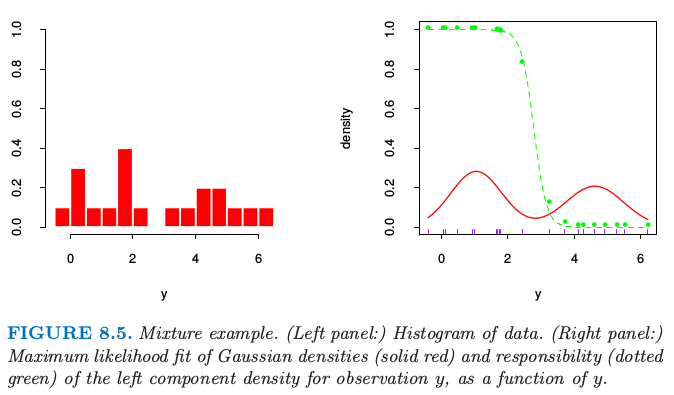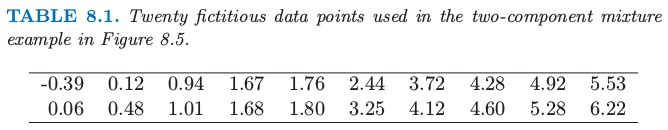weiya 注：

weiya 注：无界的似然函数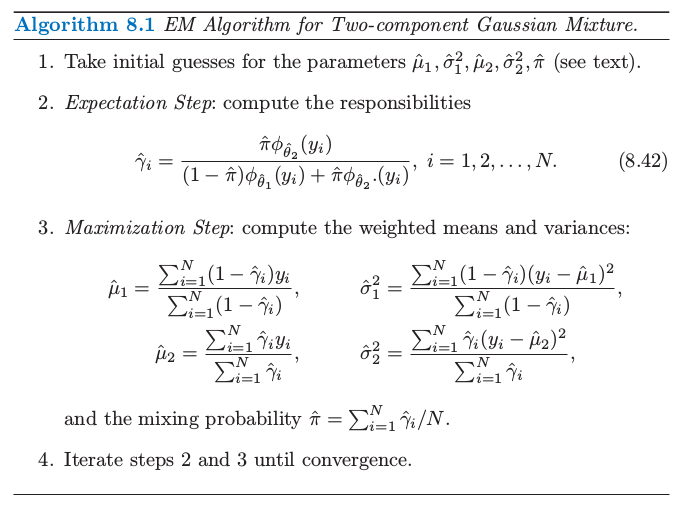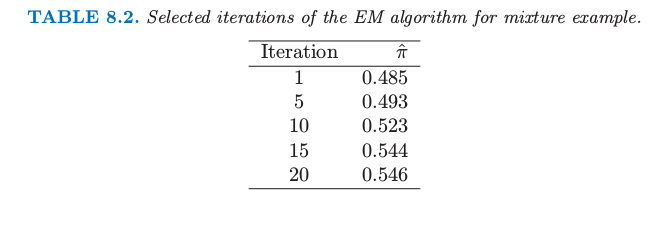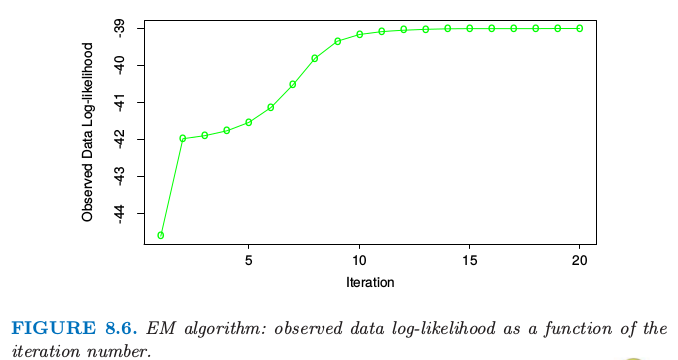weiya 注: Fig. 8.6 + Tab. 8.2

广义 EM 算法¶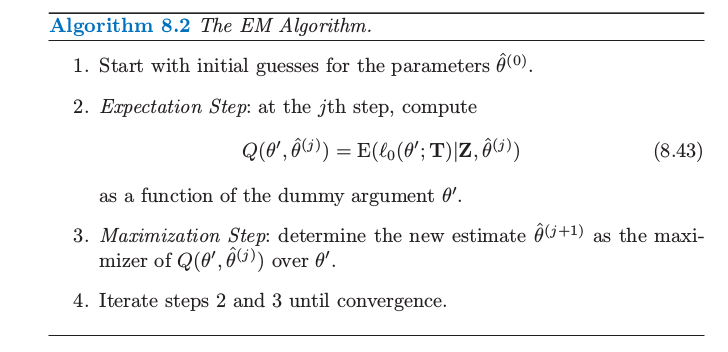Ex. 8.1

Ex. 8.7

EM 作为一个最大化-最大化的过程¶

\eqref{8.46} 式对所有 $\theta$ 都成立，包含 $\theta=\theta’$．

EM 算法可以看成 $F$ 关于 $\theta’$ 和 $\tilde P(\mathbf Z^m)$ 的联合最大化，通过固定一个变量来最大化另外一个变量．固定 $\theta’$ 来对 $\tilde P(\mathbf Z^m)$ 最大化可以证明是 练习 8.2）．

weiya 注：Ex. 8.2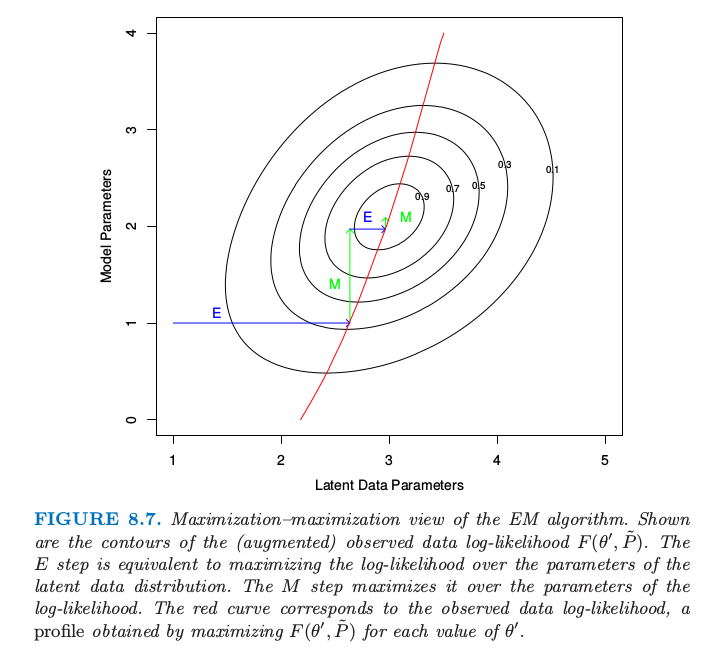EM 算法的这个角度导出了 轮换最大化过程 (alternative maximization procedure)．举个例子，虽然不需要一次性对所有潜在数据参数进行最大化，但是可以每次最大化其中的一个，通过在步骤 M 来 轮换 (alternate)

weiya 注